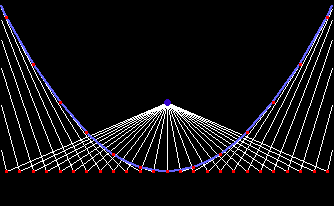# Pedal Curve

## History

From Robert Yates:

The idea of positive and negative pedal curves occurred first to Colin Maclaurin in 1718; the name “pedal” is due to Terquem. The theory of Caustic Curves includes Pedals in an important role: the orthotomic is an enlargement of the pedal of the reflecting curve with respect to the point source of light (Quetelet, 1822). (See Caustics.) The notion may be enlarged upon to include loci formed by dropping perpendiculars upon a line making a constant angle with the tangent, namely: pedals formed upon the normals to a curve.

## Description

Pedal and negative pedal are methods of deriving a new curve based on a given curve and a point.

Step by step description for positive pedal:

1. Given a curve and a fixed point O.
2. Draw a tangent at any point P on the curve.
3. Mark a point Q on this tangent so that line PQ and line OQ are penpendicular.
4. Repeat step (2) and (3) for every point P on the curve. The locus of Q is the pedal of the given curve with respect to point O.A pedal of a sine curve with respect to a point below the curve. This is one of the curves shown in the figure at the top of this page.

Pedal and negative pedal are inverse concepts. Negative pedal of a curve C can be defined as a curve C' such that the pedal of C is C'.

Step by step description for negative pedal:

1. Give a curve and a fixed point O.
2. Draw a line from O to any point P on the curve.
3. Draw a line perpendicular to line OP and passing P.
4. Repeat step (2) and (3) for every point P on the curve. The envelope of lines is the negative pedal of the given curve with respect to point O.The pedal of a parabola with respect to its focus is a line. The negative pedal of a line is a parabola.

## Formula

The pedal of a parametric curve {xf[t],yf[t]} with respect to point {a,b} is:

```{(xf'[t]^2 a + yf'[t]^2 xf[t] + xf'[t] yf'[t] (b - yf[t]))/(xf'[t]^2 + yf'[t]^2),
(yf'[t]^2 b + xf'[t]^2 yf[t] + xf'[t] yf'[t] (a - xf[t]))/(xf'[t]^2 + yf'[t]^2)}
```

## Properties

### Curve relations by pedal

Base Curve Pedal Point Pedal Curve
line any point point
circle any point limacon of Pascal
circle on circumference cardioid
parabola on directrix strophoid
parabola center of directrix right strophoid
parabola reflection of focus
by directrix
trisectrix of Maclaurin
parabola vertex cissoid of Diocles
parabola focus line
ellipse, hyperbola focus circle
Tschirnhausen's cubic focus of pedal? parabola
cissoid of Diocles focus cardioid
cardioid cusp Cayley's Sextic
deltoid cusp simple folium
deltoid vertex double folium
deltoid center trifolium
deltoid on the curve unsymmetric double folium
epicycloid center rose
hypocycloid center rose
sinusoidal spiral pole sinusoidal spiral
equiangular spiral pole equiangular spiral
involute of a circle center of circle Archimedean spiral

## Related Web Sites

Robert Yates: Curves and Their Properties .